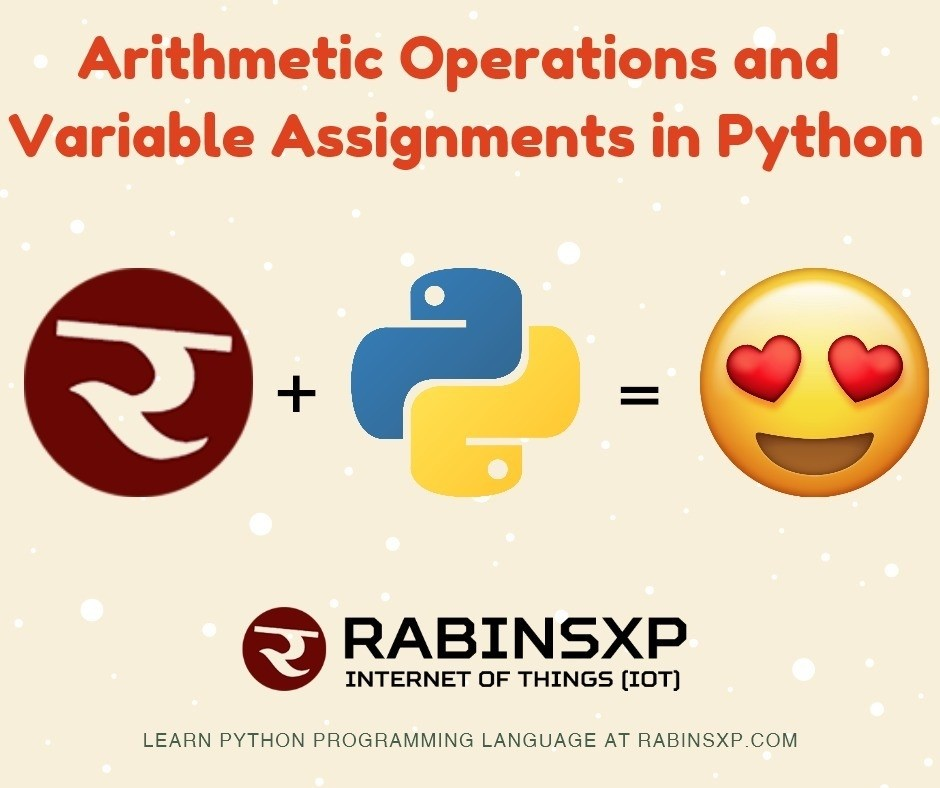Arithmetic Operations and Variable Assignments in Python

In this Python at RabinsXP, we are going to learn about the numbers, how to use them (arithmetic operations) and assigning labels to the variable. This article will cover the topics like types of numbers, basic arithmetic operations, division and assignment of the object.

Number Types

Integers: Integers are just whole numbers, positive or negative. For example, 1 and -1.

Float: Floating point numbers in Python are notable because they have a decimal point in them, or use an exponential (e) to define the number. For example, 2.0 and -2.1 are examples of floating point numbers. 4E2 (4 times 10 to the power of 2) is also an example of a floating point number in Python.

Basic Arithmetic Operations in Python

• Addition e.g. 3+4
• Subtraction e.g. 3-4
• Multiplication e.g. 2*4
• Division e.g. 6/4
• Powers e.g. 3**4
• Roots e.g. 5**0.6
• Order of operation (BODMAS rules followed)  e.g. 3 + 11 * 11 + 4
•  Specifying orders e.g. (3+11) * (11+4)

Variable Assignments

After knowing about the numbers lets move towards assigning names and creating variables in Python.

An equal sign (=) is used to assign labels to the variables with no need to specify the keyword var.

This goes like this if we want to create an object called ‘num’ and assign it with value 10 ie. num=10.

This means each time we call ‘num’, it returns the value number 10.

• Assign object e.g. num = 10
• Add objects e.g. num+num
• Reassign object  e.g.  num = 15

On reassigning the value 15 on the ‘num’, the previously stored (num=10) in the stack will be replaced and next time we call ‘num’ will result in number 15 (not the number 10). ie. we can overwrite variable names multiple times in Python.Arithmetic Operations and Variable Assignments in Python // RabinsXP

Python Rules

Listed below are the simple rules we need to follow when creating the new labels.

• Avoid starting an object name with a number.
• Whitespaces or simply space is not allowed. However, we can start using an underscore (_) instead.
• Symbols like ‘”,<>/?|\()!@#\$%^&*~-+ should not be used.
• It is suggested to use lowercase and write user-friendly names.

User-friendly object names later help in debugging logical errors others than the code error.

Conclusion

We just learned some basic arithmetic operations and rules of assigning the variables names in Python. This concludes the end of ‘Arithmetic Operations and Variable Assignments in Python’.

Next, in the next Python programming series (Python at RabinsXP), we will learn about Strings in Python.